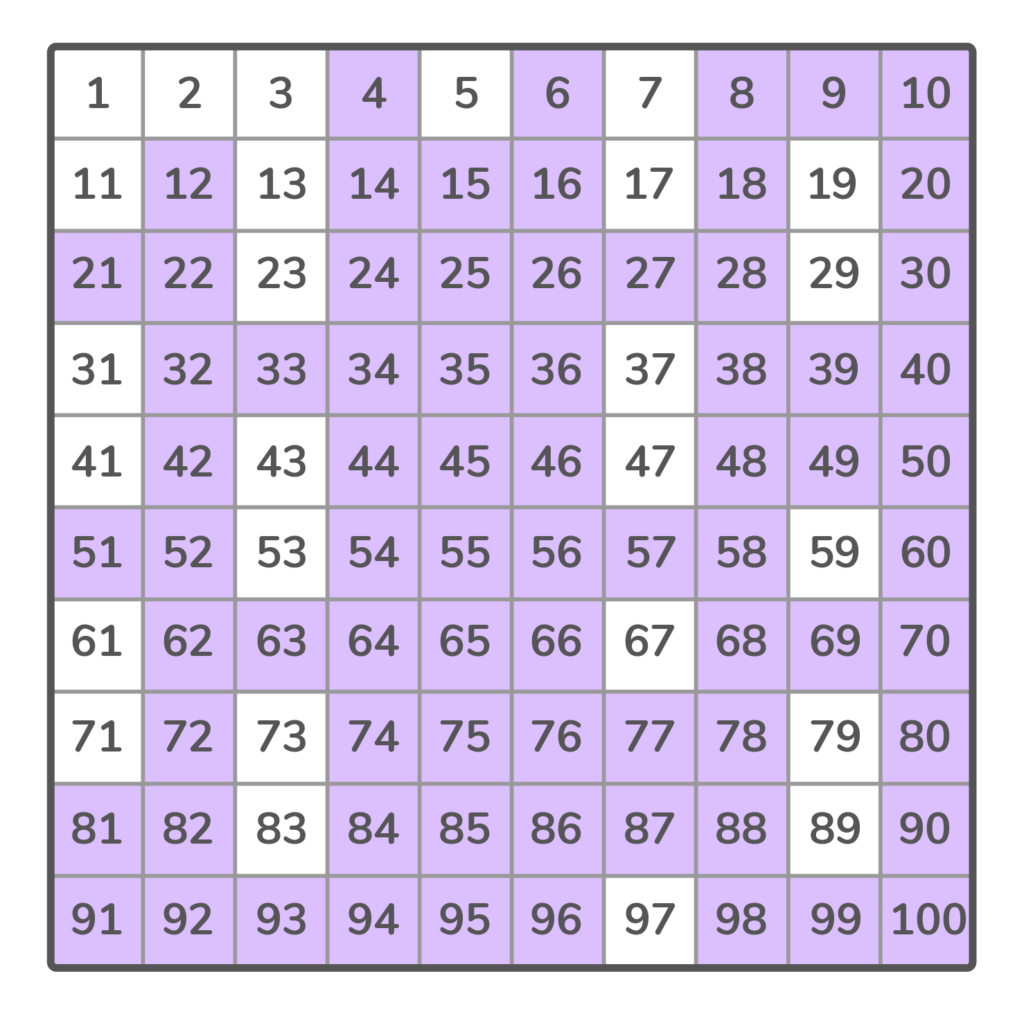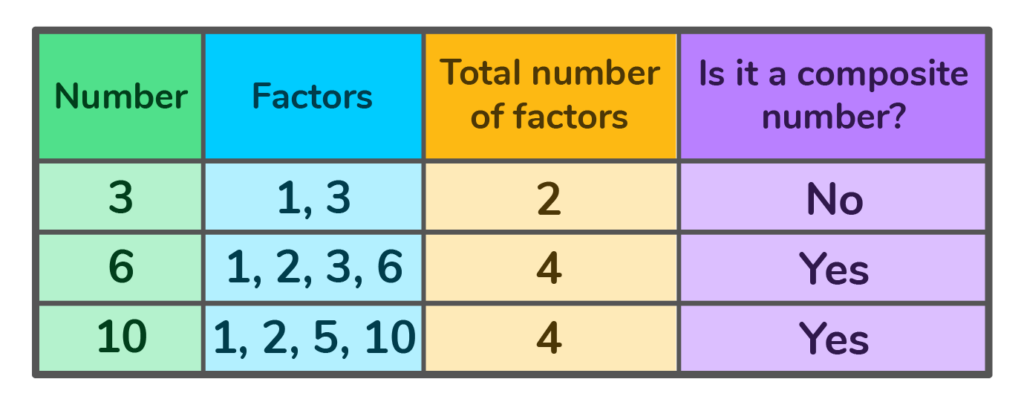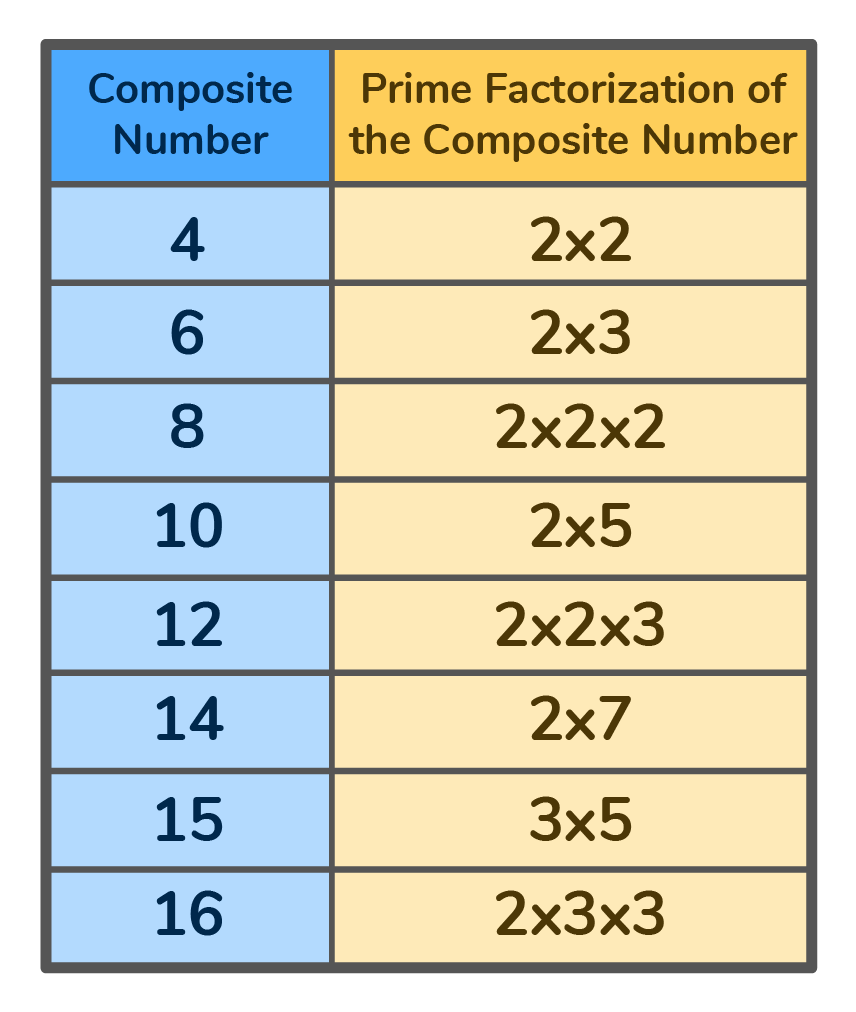# What is a composite number?

Learn the definition of composite numbers, discover how we use them in maths and check out a list of composite numbers from 1 to 100!Author
Amber WatkinsPublished
November 14, 2023# What is a composite number?

Learn the definition of composite numbers, discover how we use them in maths and check out a list of composite numbers from 1 to 100!Author
Amber WatkinsPublished
Nov 14, 2023# What is a composite number?

Learn the definition of composite numbers, discover how we use them in maths and check out a list of composite numbers from 1 to 100!Author
Amber WatkinsPublished
Nov 14, 2023Key takeaways

• Composite numbers include all natural numbers that have more than two factors
• Composite numbers are the opposite of prime numbers which only have two factors — one and itself
• The first 10 composite numbers on a number line are 4, 6, 8, 9, 10, 12, 14, 15, 16 and 18

In maths, composite numbers make up a large part of the natural numbers that we know and use. But what exactly are composite numbers?

Composite numbers include all natural numbers that have more than two factors.• 6 is a composite number because it has more than two factors: 1, 2, 3 and
• 2 is not a composite number because it only has two factors, 1 and itself

Unlock unlimited maths questions

Put your learning into practice with fun exercises + games that are proven to boost ability!

## Composite number definition

Composite numbers can be defined as any positive integers or natural numbers that have more than two factors.

These factors usually include 1 and itself, along with at least one other value. In other words, composite numbers are the opposite of prime numbers, numbers that only have two factors.

## Examples of composite numbers

The list of composite numbers begins with the natural number 4.

The first 10 composite numbers are 4, 6, 8, 9, 10, 12, 14, 15, 16, 18. What makes these numbers composite?

They all have more than two factors! Factors are two numbers you multiply to get an answer.

For example: What are the factors of 6?

1 x 6 = 6

2 x 3 = 6

So 1, 2, 3 and 6 are all factors of 6.

## Composite numbers from 1 to 100

Here are all the composite numbers from 1 to 100.## Explore composite numbers with DoodleMaths

Meet DoodleMaths, the award-wining maths app that’s proven to boost a child’s rate of progression in as little as six weeks!

Filled with fun, interactive questions covering the primary curriculum, it creates each child a unique work programme tailored to their needs, building the confidence and skills in maths. Try it for free today!## Types of composite numbers

There are two types of composite numbers that you’ll see on a number line:

1. Odd composite numbers
2. Even composite numbers

### Odd composite numbers

Odd composite numbers are any odd number that has more than two factors. This includes all positive odd numbers that are not prime numbers

The list of odd composite numbers includes 9, 15, 21, 25, and 35

Can you use the composite numbers list (from 1 to 100) to name the next three odd numbers in the above sequence?

### Even composite numbers

Even composite numbers are any even numbers that have more than two factors. This includes all positive even numbers that are not prime numbers.

The list of even composite numbers includes 4, 6, 8, 10, and 12

Can you use the composite numbers list (from 1 to 100) to name the next three numbers in the above sequence?

## More examples of composite numbers## What's the difference between prime numbers and composite numbers?

• The difference between prime numbers and composite numbers is the number of factors they have
• Prime numbers only have two factors, while the composite number definition states that composite numbers have more than two factors
• A number cannot be a prime number and a composite number

## Properties of composite numbers

• All composite numbers are divisible by 1 and itself
• All composite numbers are evenly divisible by its factors
• All composite numbers have more than two factors
• All composite numbers are divisible by prime numbers

## How to find composite numbers

When we look at a number line, it’s made up of prime and composite numbers. How can we identify which numbers are composite numbers?

### Steps to find the composite number

1. Find all the factors of a given number
2. If the number of factors is more than two, the number is a composite number
3. If the number of factors is only two, the number is a prime number!

### Find the composite number by prime factorisation

All composite numbers are divisible by prime numbers

Prime factorisation is the process of finding all the prime numbers that can be multiplied to equal the composite number.

For example: the prime factorisation for the composite number 20 is 2 x 2 x 5

The numbers 2 and 5 are both prime numbers, so we can use them to factor the number 20.

### Steps to factor a composite number by prime factorisation

1. Choose a prime number to begin multiplying
2. Continue by choosing other prime numbers to multiply until the multiplied total equals the composite number you’re factoring

## The prime factorisation of the first 10 composite numbers## Composite number practice questions

Click on the boxes below to see the answers!

Yes, 10 is a composite number because it has more than two factors! 1, 2, 5, and 10.

No, it’s not a composite number because it only has two factors (1 and 3). Since 3 only has two factors, it’s considered a prime number.

No, a number can not be both prime and composite. A prime number only has two factors.

According to the composite number definition, a composite number has more than two factors. The number of factors clearly identifies a number as either prime or composite, not both.

The first 10 composite numbers are 4, 6, 8, 9, 10, 12, 14, 15, 16, 18.

The smallest composite number is the natural number 4. The factors of 4 are 1, 2, and 4. It’s the first natural number that has more than two factors.

No, all even numbers are not composite. The number 2 is an even number. However, it only has two factors: 1 and 2.

Because the number 2 only has two factors it’s a prime number, not a composite number.

No, the number 7 is not a composite number. The number 7 only has two factors: 1 and itself. Because the number 7 only has two factors it’s a prime number, not a composite number.

No, zero is not a composite number. The number zero is neither prime nor composite.

Composite numbers are all natural numbers or positive integers that have more than two factors. A composite number can be odd or even, but never prime. To find a composite number, you must find all the factors to see if there are more than two.Lesson credits

Amber Watkins

Amber is an education specialist with a degree in Early Childhood Education. She has over 12 years of experience teaching and tutoring elementary through college level math. "Knowing that my work in math education makes such an impact leaves me with an indescribable feeling of pride and joy!"

Amber Watkins

Amber is an education specialist with a degree in Early Childhood Education. She has over 12 years of experience teaching and tutoring elementary through college level math. "Knowing that my work in math education makes such an impact leaves me with an indescribable feeling of pride and joy!"

## Related posts

Rational vs irrational numbers

Explore the differences between rational and irrational numbers

What is standard form?

Discover how standard form helps us represent numbers and equations clearly

What is place value?

Learn all about place value and take a look at some examples!

# Are you a parent, teacher or student?

Are you a parent or teacher?

## Hi there!

Book a chat with our team